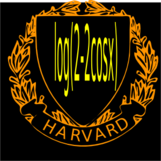Birkhoff sum project 2010
Motivation
Motivation: Birkhoff Sums Over Golden Rotation
Office: SciCtr 434
 Why do we look at this particular sum? Folkert got to such sums while working on Herman rings and I encountered such Birkhoff sums with John Lesieutre when working on some KAM (Kolmogorov-Arnold-Moser) problems. The invariant curve problem for twist maps reduces to the problem to find smooth function q satisfying ``` F(q,c) = q(x+alpha) - 2 q(x) + q(x-alpha) + c V(q(x)) = 0 ``` This is an implicit function theorem problem because for c=0, we have the solution q(x)=x. How does q continue if c becomes positive? This is a degenerate problem because Fq(q,c) the operator ```L f = f(x+alpha) + f(x-alpha) - 2 f(x) ``` which is noninvertible on L2(T). Its Fourier transform on l2 is diagonal with entries g(n alpha) and the logarithm of the determinant of the restriction to the first n Fourier coefficients is just the Birkhoff sum we study log(det(L)) = Sn. The growth of Sn depends on arithmetic properties of the rotation number alpha. The best results are obtained if alpha is the golden mean. The golden curve is the last curve which breaks up when deforming the parameter c. There are other motivations. We mention primarily the complex quadratic map T(z,w) = (c z,w(1-z)) where c=exp(2pi i alpha). The orbit (zn, wn) of (c,0) satisfies log|wn| = Sn. For |z|<1, there is an attractor, which can be seen here. One can also see Sn as the real part of the complex vortex velocity field of an n vortex ring placed on the unit circle exp(2Pi i k alpha).